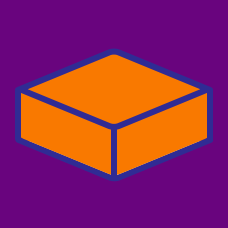Geometry

Surface Area: Level 1 ChallengesThe red figure at the right is made of two hemispheres of equal radius. The blue cylinder at the left has the same radius and height as the red figure.

Which shape has a larger surface area?

Note: Both figures are capped on the top and bottom by circles.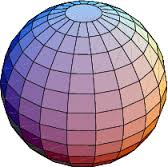If the diameter of a sphere is decreased by 25%, then by what percentage does its surface area decrease?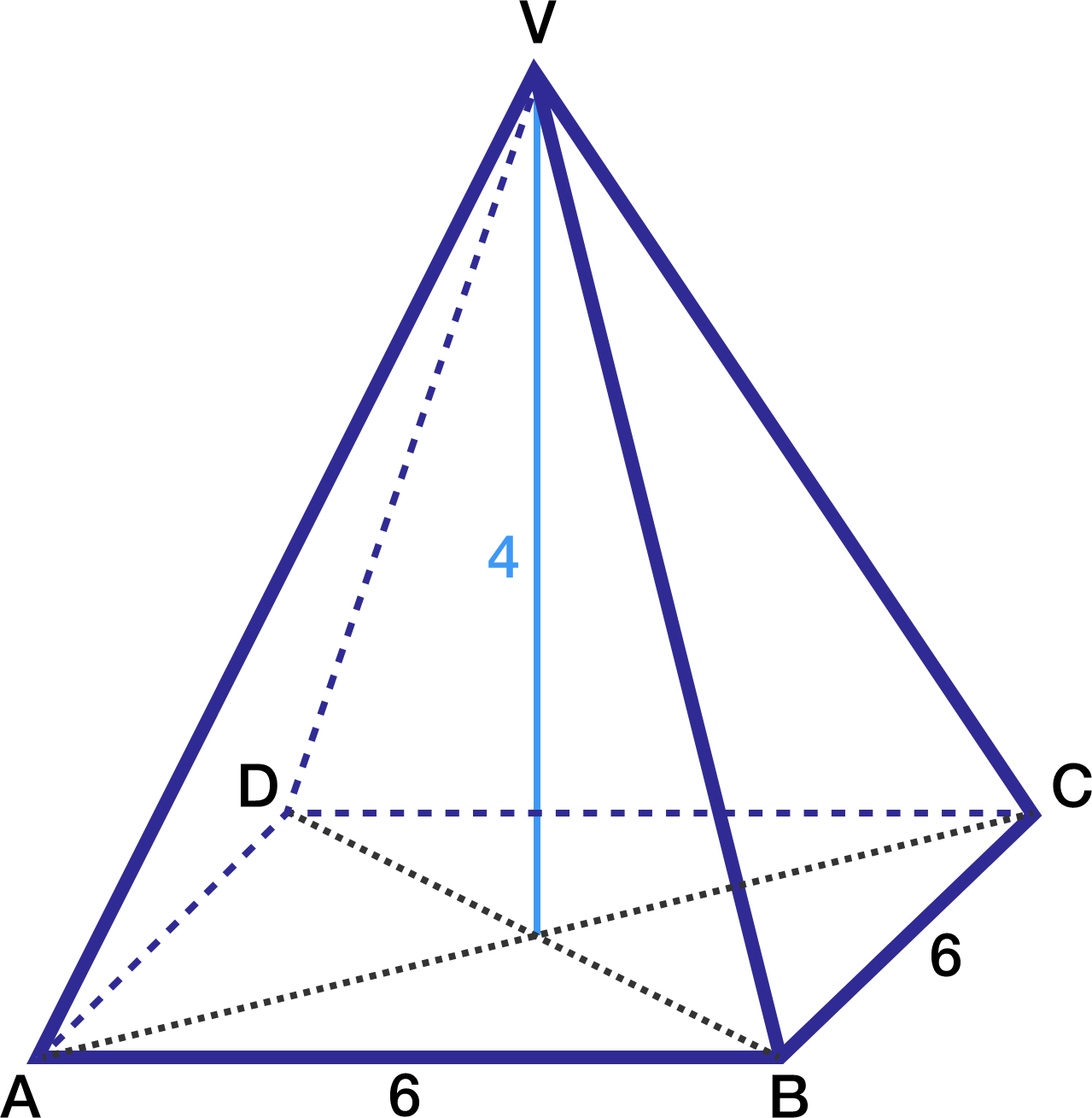Consider a pyramid with a square base of side length 6 m. The sides of the pyramid are identical isosceles triangles that meet at a common point directly above the center of the base. This point is exactly 4 m above the center.

The area of the square base is clearly $6 \times 6 = 36\text{ m}^2$. What is the total area of the 4 triangular sides, in square meters?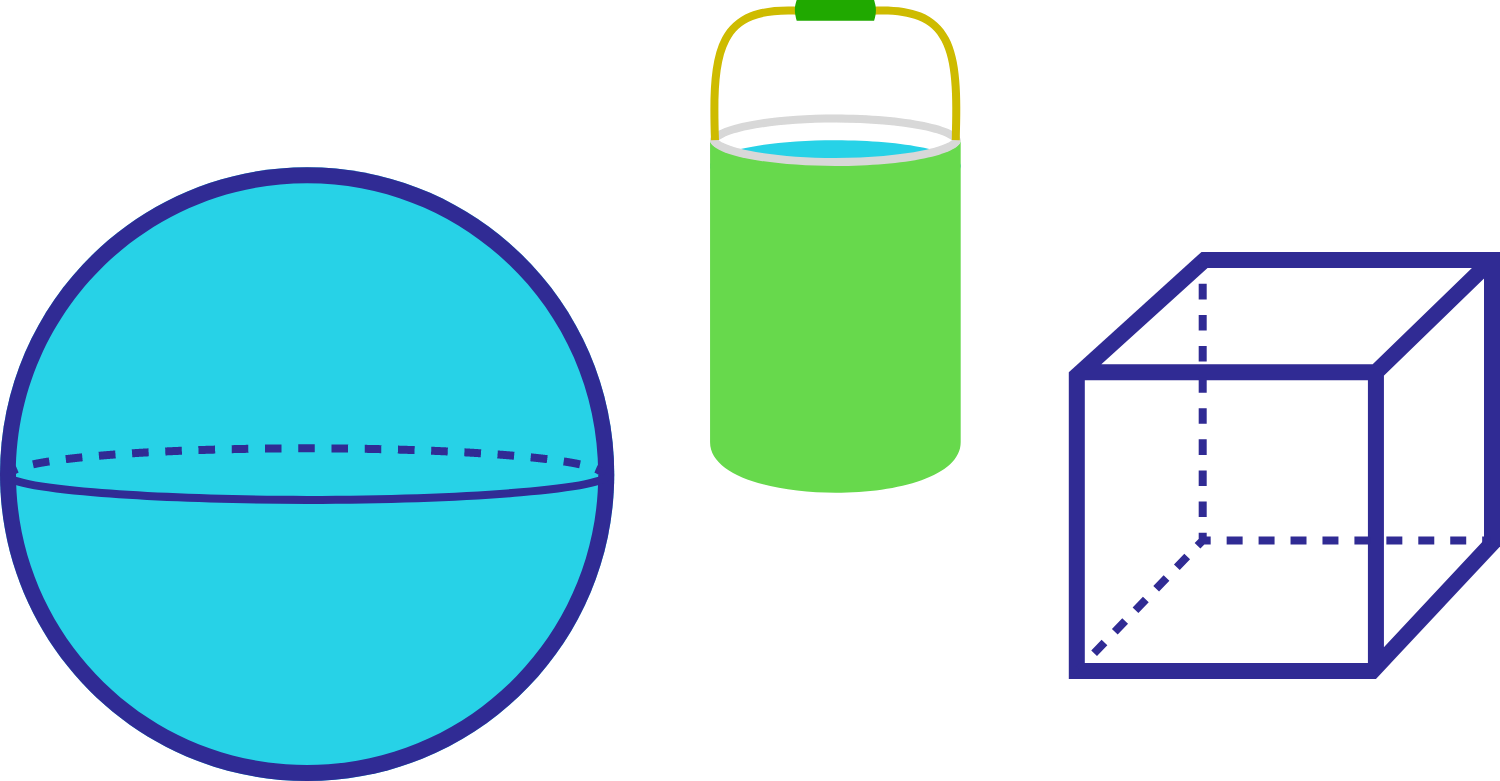You have bought a kind of paint that could paint 50 $\text{m}^{2}$ per quart. Then you used approximately 6.28 quarts of paint to color the surface of a sphere. Now you wish to paint a cube that has a side length the same as the sphere's radius. How much paint do you need, in quarts?

(P.S. use 3.14 as an approximation for pi., and $\text{m}$ means meter.)

Three cubes of side length 1 are joined together (side by side) to form a cuboid, what is the ratio of the surface areas of one of the cubes to the cuboid?

The answer is of the form $\dfrac ab$, where $a$ and $b$ are coprime positive integers.

Submit the answer as $a+b$.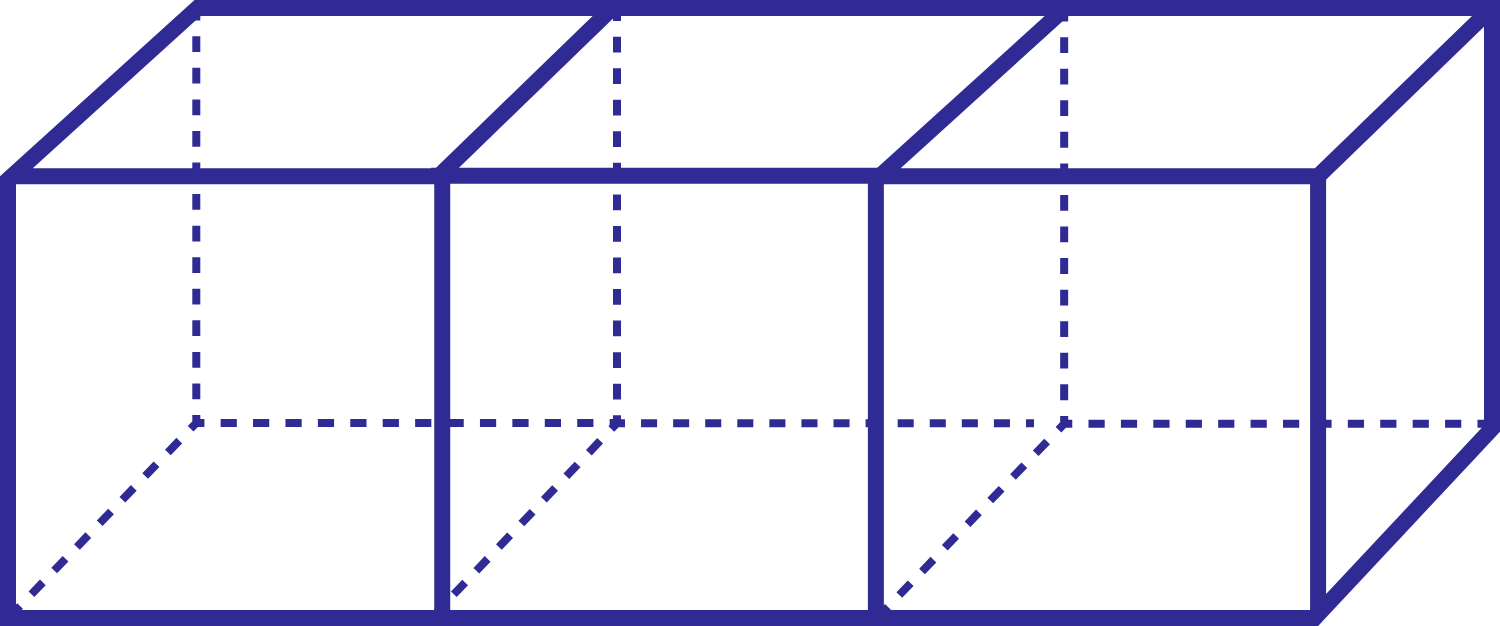×